# 二次方程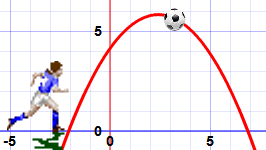## 一般形式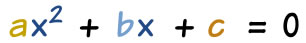a、b 和 c 是已知值，a 不能是 0。 "x" 是 变量 或未知数（我们还未知其值）。

 2x2 + 5x + 3 = 0 在这例子， a=2、b=5 和 c=3 x2 − 3x = 0 这个有点巧妙： a 在哪里？其实，a=1，我们通常不这样写的： "1x2" b = -3 c 呢？c=0，所以不写出来。 5x − 3 = 0 哎呀！ 这个 不是 二次方程：没有 x2 换句话说，a=0，就不是二次式）

## 隐藏的二次方程！

ax2 + bx + c = 0

x2 = 3x − 1 把所有的项移到左边 x2 − 3x + 1 = 0 a=1、b=−3、c=1
2(w2 − 2w) = 5 展开（拆开 括号），

2w2 − 4w − 5 = 0 a=2, b=−4, c=−5
z(z−1) = 3 展开，把3移到左边 z2 − z − 3 = 0 a=1, b=−1, c=−3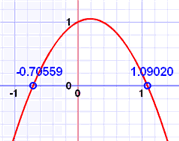• 它画的图，和
• 方程的解（叫"根"）。

## 怎样解方程？## 关于二次公式

### 正/负± 的意思是有两个答案：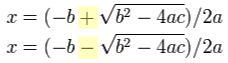这是为什么有两个答案。### 判别式

• b2 - 4ac 是正数时，方程有两个
• 当判别式是零时，方程只有一个实解（两个相同的答案）
• 当判别式是负数时，方程有两个

### 例子：解 5x² + 6x + 1 = 0

 系数是： a = 5、b = 6、c = 1 二次公式： x = −b ± √(b2 − 4ac) 2a 代入 a、b 和 c： x = −6 ± √(62 − 4×5×1) 2×5 解： x = −6 ± √(36 − 20) 10 x = −6 ± √(16) 10 x = −6 ± 4 10 x = −0.2 或 −1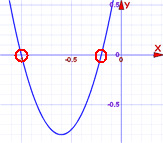答案： x = −0.2 或 x = −1   如图。

 检验 -0.2: 5×(−0.2)² + 6×(−0.2) + 1 = 5×(0.04) + 6×(−0.2) + 1 = 0.2 − 1.2 + 1 = 0 检验 -1: 5×(−1)² + 6×(−1) + 1 = 5×(1) + 6×(−1) + 1 = 5 − 6 + 1 = 0

## 复解？

### 例子：解 5x² + 2x + 1 = 0

 系数是： a = 5, b = 2, c = 1 注意： 判别式 是负数： b2 − 4ac = 22 − 4×5×1 = -16 用二次公式： x = −2 ± √(−16) 10 -16 的平方根是 4i (i 是 √-1，去 虚数 来了解更多） 结果： x = −2 ± 4i 10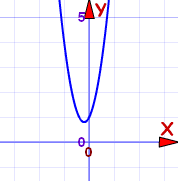答案： x = −0.2 ± 0.4i   图不经过 x轴。这就是为什么解是复数。

## 总结

• 二次方程的一般形式：ax2 + bx + c = 0
• 二次方程可以被 因式分解
• 二次公式：x = −b ± √(b2 − 4ac) 2a
• 当判别式(b2−4ac)为：
• 正数时，方程有 2 个实解
• 零时，方程有一个实解
• 负数时，方程有 2 个复解

（难题： 1 2 3 4 5 6 7 8